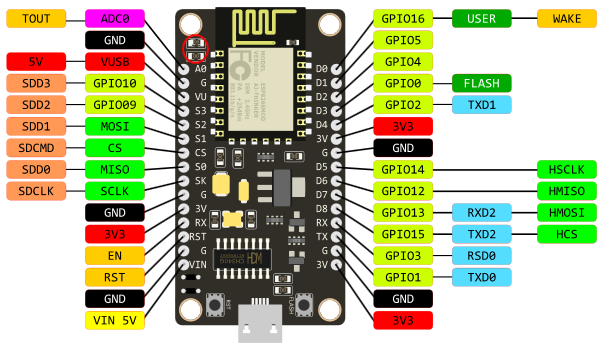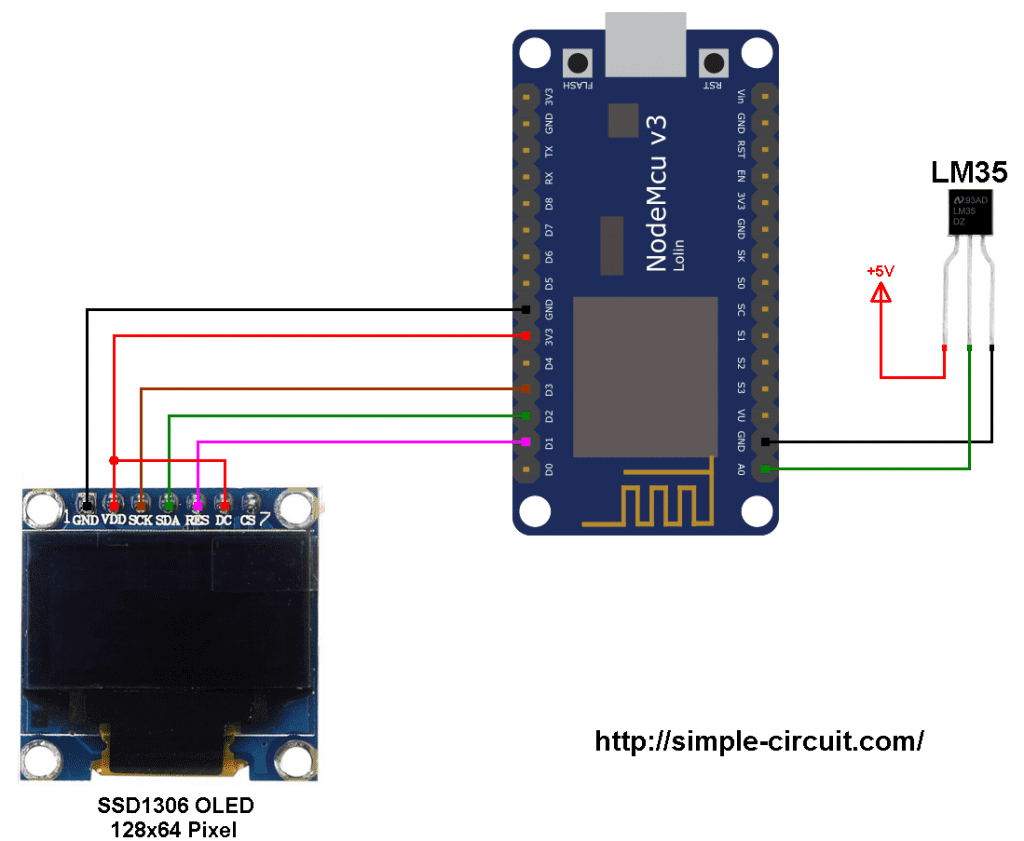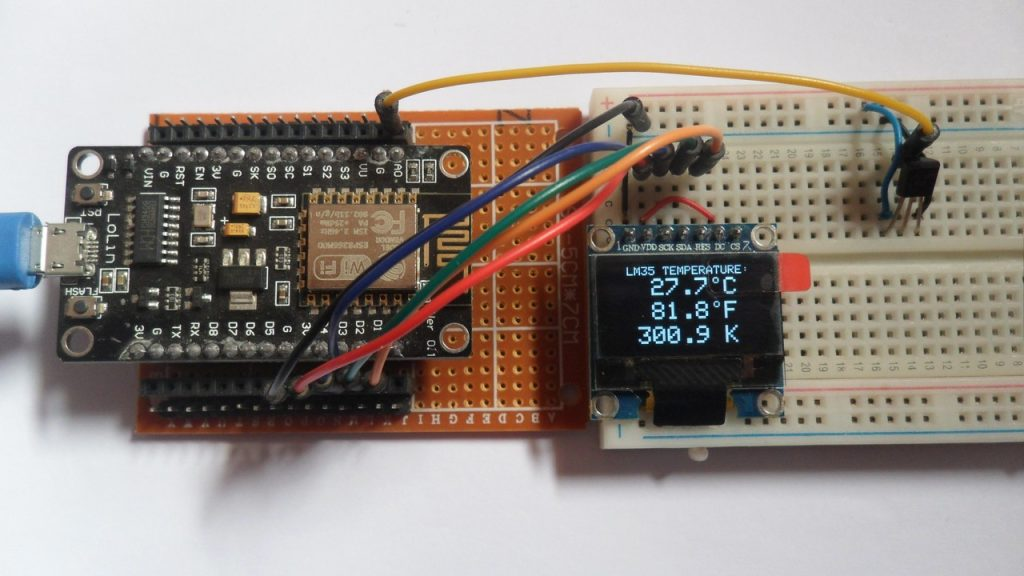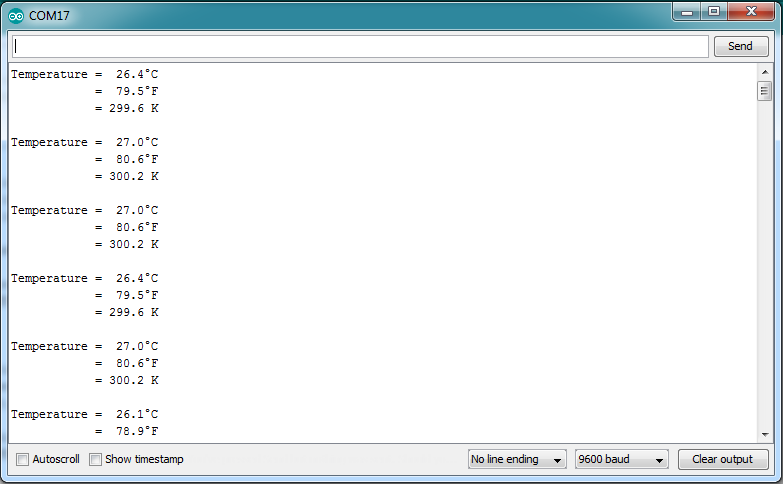# Interfacing NodeMCU with LM35 temperature sensor

This tutorial shows how to interface ESP8266 NodeMCU development board (ESP12-E module) with LM35 analog temperature sensor and SSD1306 OLED display.
In this project the SSD1306 OLED display (128×64 pixel) is used to display environment temperature in degree Celsius, Kelvin and degree Fahrenheit.
Also, the 3 quantities of the temperature are printed on Arduino IDE serial monitor.

The SSD1306 OLED display used in this project is configured to work in I2C mode, some SSD1306 OLED boards may require a small hardware modifications (to select between SPI mode and I2C mode) such as soldering, placing jumpers …

To see how to interface ESP8266 NodeMCU with SSD1306 OLED display, visit the following post:
ESP8266 NodeMCU interfacing with SSD1306 OLED

The LM35 temperature sensor is a three pin device (VCC, OUT and GND) with an output voltage linearly related to Centigrade temperature. Since the LM35 output varies with dependent to the temperature, we need an ADC (Analog-to-Digital Converter) module to measure this voltage. The NodeMCU microcontroller (ESP8266EX) has one ADC module with 10-bit resolution.

The LM35 output has linear +10mV/°C scale factor means the following:
If the output voltage =   10mV —> temperature =   1°C
If the output voltage = 100mV —> temperature = 10°C
If the output voltage = 200mV —> temperature = 20°C
If the output voltage = 370mV —> temperature = 37°C
and so on.

LM35 Futures (from datasheet):

• Calibrated Directly in ° Celsius (Centigrade)
• Linear + 10 mV/°C Scale Factor
• 0.5°C Ensured Accuracy (at +25°C)
• Rated for Full −55°C to +150°C Range
• Suitable for Remote Applications
• Low Cost Due to Wafer-Level Trimming
• Operates from 4 to 30 V
• Less than 60-μA Current Drain
• Low Self-Heating, 0.08°C in Still Air
• Nonlinearity Only ±¼°C Typical
• Low Impedance Output, 0.1 Ω for 1 mA Load

The ADC module converts analog data into digital data. The ESP8266EX MCU has a 10-bit ADC module with one analog channel. Negative and positive references of the ADC module are 0V (GND) and 1.0V respectively. That means a 0V is represented by 0 and 1.0V (or higher but we shouldn’t apply more than that) is represented by 1024.

LoLin version of the NodeMCU board analog input is A0 (or ADC0), it’s connected to the ESP8266EX microcontroller through voltage divider of 2 resistors (220k and 100k ohm) as shown in the image below (red circle).
With the built-in voltage divider, the NodeMCU analog channel supports inputs from 0 to 3.3V.Also, using the LoLin NodeMCU we can supply the LM35 sensor with 5V that comes from pin 5V (or VUSB).

Hardware Required:

• ESP8266 NodeMCU development board
• SSD1306 OLED display with 128×64 pixel resolution
• LM35 temperature sensor   —->   datasheet
• micro USB cable (for programming and powering the circuit)
• 5V source (optional)
• Jumper wires

Interfacing NodeMCU with LM35 sensor circuit:
Project circuit schematic diagram is shown below.

The LM35 sensor has 3 pins (from left to right):
Pin 1 is power supply pin, connected to external 5V power source positive terminal (don’t forget to connect its negative terminal to any of the NodeMCU ground pins).
The NodeMCU LoLin V3 has a pin that provides 5V, it can be used to supply the LM35 sensor. Otherwise, connect it to any 3.3V pin (not recommended because voltage range is from 4V to 36V).
Pin 2: output pin, connected to pin A0 (ADC0),
Pin 3: GND (ground), connected to NodeMCU GND pin.The SSD1306 OLED display is connected to the NodeMCU board as follows:
SSD1306 OLED GND goes to NodeMCU GND pin,
SSD1306 OLED VDD to NodeMCU 3V3,
SSD1306 OLED SDA pin (serial data) to NodeMCU pin D2 (GPIO4),
SSD1306 OLED SCK pin (serial clock) to NodeMCU pin D3 (GPIO0),
SSD1306 OLED RES pin (reset) to NodeMCU pin D1 (GPIO5).

The SSD1306 OLED display DC pin is connected to VDD (3.3V) which means I2C slave address of the device is 0x3D. If the DC pin is connected to ground (GND) then the I2C slave address becomes 0x3C.

Interfacing NodeMCU with LM35 sensor circuit:
The following Arduino code requires 2 libraries from Adafruit Industries:
The first library is a driver for the SSD1306 OLED display which can be installed from Arduino IDE library manager (Sketch —> Include Library —> Manage Libraries …, in the search box write “ssd1306” and install the one from Adafruit).

The second library is Adafruit graphics library which can be installed also from Arduino IDE library manager.

The same thing for the other library file.

In the code there are total of 3 libraries, they’re included in the code as follows:

Definition of the SSD1306 OLED reset pin (RES) and initialization of its library are as shown below:

Reading analog voltage using ADC module gives a number between 0 and 1024 (10-bit resolution), 0V is represented by 0 and 3.3V is represented by 1024. Converting back the ADC digital value is easy, we can use the following equation:

This voltage (in milliVolts) represents the temperature value in tenths degree Celsius (output value of “273” equals 27.3 °Celsius).

The temperature in tenths degree Fahrenheit =  (tenth °Celsius) x 9/5 +320 (because: °F = °Cx9/5 + 32) and the temperature in tenths Kelvin = (tenth °Celsius) + 2732 (because: K = °C + 273.16).

To get the actual value of each quantity we’ve to divide it by 10. The line below shows an example for temperature in Kelvin:

We get the first 3 digits by dividing the tenths value by 10, and the tenths number (number after the decimal point) of the actual temperature value is equal to the reminder of that division (tenths value % 10).

The resolution of this thermometer is about 0.3°C.

Full Arduino code:

The following picture shows a protoboard circuit of the project:Arduino IDE serial monitor result:This site uses Akismet to reduce spam. Learn how your comment data is processed.